## Year 3 mental arithmetic: sets 53 and 54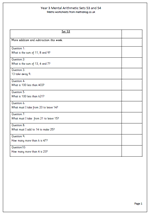I continue on with the weekly sets of mental arithmetic for Year 3. This week the questions concentrate on addition and subtraction, including adding three numbers ‘in your head’. Both the first two questions are designed so that pairs that make 10 can be spotted e.g. 11 + 8 + 9, where the easiest way to do this is to firstly add the 11 and 9 to make 20.

Children need to listen carefully to the questions to make sure that they carry out the right calculation. For example;

‘What must I add to 14 to make 25?’

Some children will here add, 14 and 25 and immediately add 14 and 25 to make 39, when they should, of course, subtract.

In a similar ay, the question:

‘How many more than 4 is 23?’

will be interpreted by some as adding 4 to 23 rather than counting on from 4 to 23.

Year 3 mental arithmetic sets 53 and 54

## Resource of the week: change from ten pence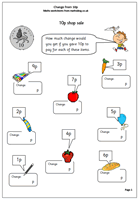Using coins is a vital part of understanding number as it provides concrete examples of numbers in action. It is a really good idea to have a set of coins that can be counted out and swapped e.g. 5 one pence coins exchanged for a 5p piece etc. Why not set up a little shop at home and take turns buying and selling items? There is an amazing amount of maths involved in this, from learning how to write numbers to counting on and back and finding two or three lots of a number. If a shop is not available why not try this page?

Thanks to urbrainy.com for this money worksheet, suitable for year 1 children.

The worksheet asks for the change needed after spending various amounts. The easiest way to work out change is to start with the amount spent and count on up to 10. Eventually it is expected that children will know, off by heart’ the answers. In other words they will know, without counting that if you spend 6p you will have 4p change.

Change from 10p

## Year 3 Mental Arithmetic: sets 51 and 52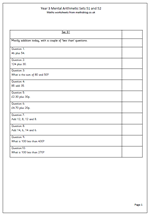This week’s year 3 mental arithmetic questions concentrate on addition, with a couple of ‘less than’ questions. Children should be getting more familiar with the various techniques they can use to answer questions ‘in their heads’.

For example:

a. it helps to look for pairs of numbers that make 10 such as 5 and 5 or 6 and 4. These pairs all need to be learnt off by heart.

b. when adding pairs of multiples of ten, use knowledge of adding single digits. If the answer to 6 + 7 is known off by heart it makes it easy to add 60 and 70.

c. when adding pounds and pence make sure the answer is using the correct units e.g. £2.70 + 20p = £2.90. (Not £2.90p)

d. when adding three or four numbers to remember that addition can be done in any order and it is often best to start with the largest number.

Year 3 mental arithmetic (sets 51 and 52)

## Resource of the Week: Year 6 Prime Factors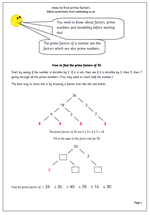The term ‘prime factor’ is one which would make many adults shudder as they try to think back to their school days. In fact, it is a term that children would probably not met until secondary school, but is now firmly incorporated into the primary curriculum.

Here we have a maths worksheet for year 6 on how to find prime factors. Before attempting this page it is necessary for children to have a good understanding of what factors are and what prime numbers are.

One of the easiest ways of finding prime factors is to use a diagram, rather like a tree diagram with the numbers at the bottom all being the prime factors. Frequently the same number is found more than once. For example the prime factors of 36 are 3, 3, 2 and 2.

Starting with the number itself, find two numbers which multiply together to make that number. For example 36 can be made by multiplying 18 by 2. 18 and 2 are both factors of 36. Repeat this process until only prime numbers are left.

A good way of checking if all the factors have been found is to multiply them and the original number should result. e.g. 3 x 3 x 2 x 2 = 36

Go to prime factors page

## More long division: 3-digits divided by 2-digits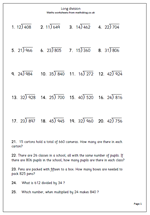One of the hardest things children try to do in primary school is long division. It is hard because it requires a good knowledge of tables,  the ability to multiply, estimate, use trial and improvement and go through several steps to reach the answer. These steps include:

e.g. 789 divided by 36

First carry out an estimate of the answer. I think 789 divided by 36 is about 20.

a. How many 36s in 78?

b. 2 x 36 is 72.    3 x 36 is 108 which is too many, so it must be 2.

c. Put the 2 in the tens column above the question.

d. Place the 72 below the 78 and subtract.

e. 78 – 72 is 6.

f. ‘Bring down’ the 9 to make 69.

g. How many 36s in 69.

h. By trial and improvement and some rough work multiplying 36 by my estimated numbers I find that 36 x 1 = 36.

i. Put the 1 in the units column above the question.

j. Place the 36 under the 69 and subtract.

k. The remainder (33) must be less than the original number you are dividing by.

Good luck with these, but many year 6 children will find them too tricky! When I was at school I used to like doing pages of these because I had learnt the method ‘off by heart’ and it was just a matter of repeating it.

Division 3 by 2

## Year 3 mental arithmetic: sets 49 and 50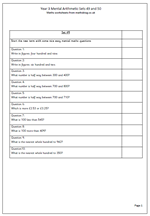We begin the summer term’s mental arithmetic papers for year 3 with a reasonably easy set of questions. The first two questions look at writing numbers in figures. e.g. ‘write in figures: four hundred and nine. ‘ Most children should be able to do this but watch out for those who are unsure about place value and write 4009.

The next two questions are about finding numbers half way between two other numbers. These are not as easy as they look. There are also questions on ‘more than’ and ‘less than’ as well as a couple of rounding to the nearest whole one hundred.

These mental arithmetic pages can be used in a variety of ways. The questions can be read out without children seeing them or the sheet can be printed out for answers to be written down. If reading them they will need to be repeated at least once and time given for the answers to be worked out. It is much harder to retain information than to have it written.

Year 3 mental arithmetic (sets 49 and 50)

## Key Stage 2 Maths SAT practice: graphs (4)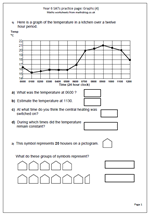This worksheet is another in our series on the types of questions that come up on the SAT papers. It is another on graphs, but quite a lot harder. The first part of the worksheet looks at a line graph showing temperatures in a kitchen over a 12 hour period.A key difference between this and other graphs is that every point on the line has a value even between the hours marked.

As well as reading times from the graph it also asks the question, ‘At what time do you think the central heating was switched on?’ This involves interpreting the question and assuming that switching on the central heating would lead to a rise in temperature. Given that it would take a little while for the radiators to warm up it would seem that 0500  would be a good answer. Times either side of this could also be given as correct.

The second part of the worksheet looks at a pictogram, where each symbol represents 20 houses. Again, this is a common type of question that children should have met many times before and should not cause any problems.

Graphs (4)

## Year 6 SATs practice: graphs (3)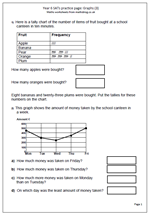Here is the next in our mini series of maths worksheets looking at the types of questions involving graphs that come up in the SAT papers. The first part of the worksheet looks at interpreting a tally chart. Children should have had plenty of practice with making and interpreting tally charts from year 2/3 onwards so this should be a very simple  question.

The second part of the worksheet is harder, with questions on a line graph. The scale goes up in hundreds and there needs to be a reasonable amount of leeway in the answers given.

This page can be found in the Year 6 maths worksheets category, under Key Stage 2 Maths SAT practice, together with other pages on number, time and shape. Also don’t forget to take a look at Year 6 Maths SAT Papers where you will find lots more questions taken from recent test papers plus ways to approach them.

Graphs (3)

## Year 6 SATs practice: Graphs (1 and 2)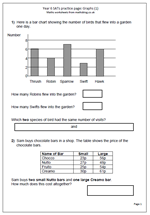It is not too long now before the latest round of Year 6 SATs comes so I thought I would put a week aside to provide worksheets to help with this.

Today I have published a page which looks at the type of question which comes up on interpreting bar charts. A bar chart shows the number of birds that flew into a garden on one day. It is very straightforward, with the numbers on the axis going up in twos.

The second part of the page looks at using a table to find information and the question asks how much two Nutto bars and one Creamo bar would cost. The first step is to find the correct prices and then work out the cost. Two bars at 27p and one at 61p can be added in several ways. For example:

The 27p can be doubled to make 54p and then the 61p added to make 115p or £1.15 or

27p can be added to 61p to make 88p and then add 27p.

The second page is similar, this time looking at a temperature graph and a frequency of throwing dice graph.

These worksheets can be found in the Year 6, Key Stage 2 Maths SAT practice category.

Graphs (1)

Graphs (2)

## Maths worksheet: Multiplying by 2, 3, 5 and 10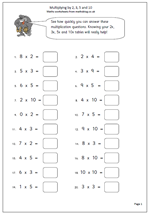Here is a straightforward maths worksheet which looks at multiplying by 2, 3, 5 and 10. Probably this page can be best used as an assessment page to see just how well these tables have been learned. They are the first four tables to be learnt and only the three times tables should cause much in the way of difficulty.

Some children do find it incredibly difficult to learn times tables so it is very worthwhile to keep coming back to them, repeating them time and time again until they become ‘second nature’.

When learning times tables it is important to say it in full and not just give the answers. For example a child might be able to recite counting on in twos: 2, 4, 6, 8, 10, 12 etc but if asked what six times two was would have to count up from two, probably using fingers to know when 6 has been reached. This is not an effective approach.

This page can be found in the Year 3 category on Calculating.